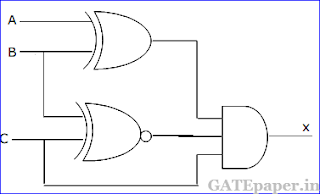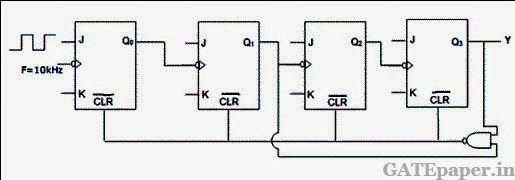### GATE 2000 ECE Video Solution on Digital Circuits (Digital Electronics)

1. The number of comparators in a 4 bit flash ADC is
a. 4
b. 5
c. 15
d. 16

2. For the logic circuit shown in the figure, the required input combination (A,B,C) to make the output X = 1 isa. 1, 0, 1
b. 0, 0, 1
c. 1, 1, 1
d. 0, 1, 1

3. In the 8085 microprocessor, the RST 6 instruction transfers the program execution to the following location
a. 30H
b. 24H
c. 48H
d. 60H

4. An 8 bit successive approximation analog to digital converter has full scale reading of 2.55 volts and its conversion time for an anlog input of 1 volt is 20 µs. The conversion time for a 2 volts input is
a. 10 µs
b. 20 µs
c. 40 µs
d. 50 µs

5. The number of hardware interrupts (which require an external signal to interrupt) present in an 8085 microprocessor are
a. 1
b. 4
c. 5
d. 13

6. The most commonly used amplifier in sample and hold circuits is
a. A unity gain inverting amplifier
b. A unity gain non inverting amplifier
c. An inverting amplifier with a gain of 10
d. An inverting amplifier with a gain of 100

7. For the logic circuit shown, the simplified Boolean expression for the output Y isa. A + B + C
b. A
c. B
d. C

8. For the 4 bit DAC shown, the output voltage Vo isa. 10 volts
b. 5 volts
c. 4 volts
d. 8 volts

9. A sequential circuit using D flip flop and logic gates is shown in figure, where X and Y are the inputs and Z is output. The circuit isa. S-R flip flop with inputs X = R and Y = S
b. S-R flip flop with inputs X = S and Y = R
c. J-K flip flop with inputs X = J and Y = K
d. J-K flip flop with inputs X = K and Y = J

10. The contents of register (B) and Accumulator (A) of 8085 microprocessor are 49H and 3AH respectively. The contents of A and the status of carry flag (CY) and sign flag (S) after executing SUB B instruction are
a. A = F1 , CY = 1 , S = 1
b. A = 0F, CY = 1, S = 1
c. A = F0, CY = 0, S = 0
d. A = 1F, CY = 1, S = 1

11. In the given figure, the J and K inputs of all the four flip-flops are made high. The frequency of the signal at output Y isa. 0.833 KHz
b. 1.0 KHz
c. 0.91 KHz
d. 0.77 KHz

12. For the CMOS monostable multivibrator of given figure, R = 50 KΩ and C = 0.01 µF, VDD = 5 volts, and the CMOS NOR gates have a threshold voltage (VT) of 1.5 volts. Vin is a trigger pulse (τp << RC) as shown in the figure.a. Plot VA1 and Vo1 as functions of time.
b. Write the equation for VR(t), for t > 0
c. Find the time period of the output pulse.

13. The operating conditions (ON = 1 and OFF = 0) of three pumps (x, y, z) are to be monitored, x = 1 implies that pump x is on. It is required that the indicator (LED) on the panel should glow when a majority of the pumps fail.a. Enter the logical values in the K map in the format shown in figure. Derive the minimal Boolean sum of products expression whose output is zero when a majority of the pumps fail.
b. The above expression is implemented using logic gates, and point P is the output of the circuit, as shown in given figure, P is at 0 volts when a majority of the pumps fails and is at 5 volts otherwise. Design a circuit to derive the LED using this output.
The current through the LED should be 10 mA and the voltage drop across it is 1 volt. Assume that P can source or sink 10 mA and a 5 volts supply is available.

14. A one bit full adder is to be implemented using 8 to 1 multiplexers (MUX).
a. Write the truth table for sum (S) and carry to the next stage (CN), in terms of the two bits (A, B) and the carry from the previous stage (CP). The truth table should be in the ascending order of (A, B, CP) i.e. (000, 001, 010..... etc).
b. Implement S and CN using 8 to 1 multiplexers.

15. The program and machine code for an 8085 microprocessor are given by
The starting address of the above program is 7FFFH. What would happen if it is executed from 8000H?Solution :